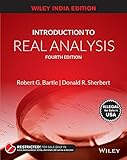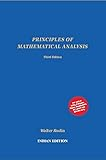# Puzzle: Three Connected Sets

🛈 This materials is useful for Unit 1 in CSIR-NET.

True or False: Let A and B be two connected subsets of a metric space. If B is a set such that A ⊆ B ⊆ C, then B is connected.
TRUE
Wrong. Taking counterexamples, let A = {1}, B = {1} U {2}, C = [1,2]. Clearly, the hypothesis is satisfied, but B is not connected.
FALSE
To prove, you need a proof. To disprove, you need a counterexample. Leave your proof below in comments!

## Recommended Books for Real AnalysisIntroduction to Real Analysis by Bartle and Sherbert (4e)Principles of Mathematical Analysis by Rudin